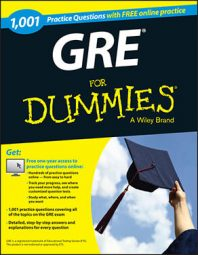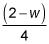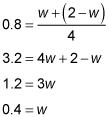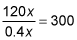##### GREThe word problems on the GRE Math test may include weighted averages, rate/time/distance, and permutations and combinations. If you're fresh out of math class, you'll probably be fine going through these quickly.

If it's been a few years since you've tackled any word problems, you may want to work these questions carefully. Shortfalls in math are in three basic categories. See whether you're prone to one of these in particular:

• Mistakes in basic math, such as not placing the decimal point correctly

• Mistakes in working the problem, such as multiplying exponents when you should be adding them

• Not knowing how to work a certain math problem, such as setting up a probability

The following practice questions will test your skills at calculating the weight of a cookie tin and the average donation needed based on existing donations.

## Practice questions

1. The total weight of a tin and the cookies it contains is 2 pounds. After 3/4 of the cookies are eaten, the tin and the remaining cookies weigh 0.8 pounds. What is the weight of the empty tin in pounds?

A. 0.2

B. 0.3

C. 0.4

D. 0.5

E. 0.6

2. A church was able to raise 75% of the total amount it needs for a new building by receiving an average donation of \$600 from each parishioner. The people already solicited represent 60% of all parishioners. If the church is to raise exactly the amount it needs for the new building, what should be the average donation from the remaining people to be solicited?

A. \$150

B. \$250

C. \$300

D. \$400

E. \$500

1. C. 0.4

Let the weight of the empty tin = w.

The weight of the cookies = (2 – w).

One quarter of the cookies weighOne quarter of the cookies + tin = 0.8.The weight of the empty tin is 0.4 pounds, Choice (C).

2. C. \$300

Let x be the total number of people the church will ask for donations.

First, calculate the amount of money already raised:

(600)(0.6x) = 360x

360x constitutes 75% of the total amount needed, so 25% = 120x.

Next, calculate the average donation needed from the remaining people:The average donation needed from the remaining parishioners is \$300, Choice (C).